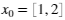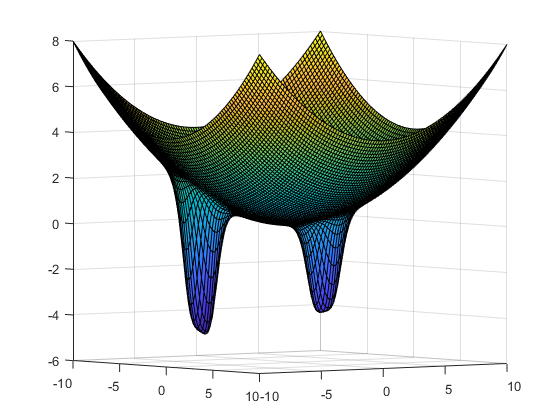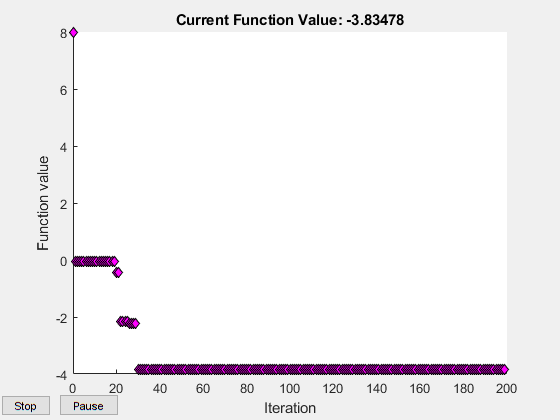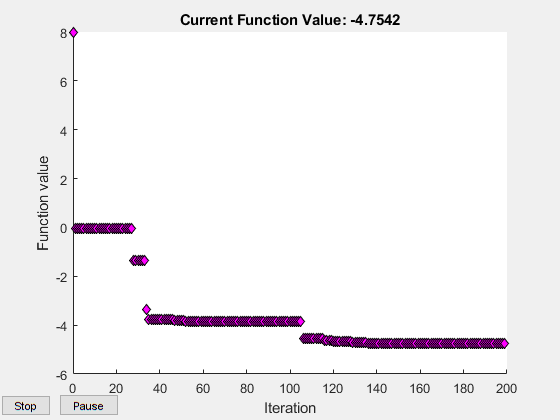Loren on the Art of MATLABTurn ideas into MATLAB

This is machine translation

Mouseover text to see original. Click the button below to return to the Original version of the page.

Surrogate Optimization for Expensive Computations

Posted by Loren Shure,

I'd like to introduce this week's guest blogger Alan Weiss. Alan writes documentation for mathematical toolboxes here at MathWorks.

Hi, folks. This post is about a new solver in Global Optimization Toolbox, surrogateopt. This solver differs from many of our previous solvers in the following ways:

• It is best suited to optimizing expensive functions, meaning functions that are time-consuming to evaluate. Typically, you use surrogateopt to optimize a simulation or ODE or other expensive objective function.
• It is inherently a global solver. The longer you let it run, the more it searches for a global solution.
• It requires no start point, just problem bounds. Of course, you can specify start points if you like.

The solver is closest in concept to a Statistics and Machine Learning Toolbox™ solver, bayesopt. Both of these solvers work by internally creating a model of the objective function, and using the internal model to attempt to find better points to evaluate. They each attempt to find a global solution, but they use different techniques to do so. They both have overhead (take noticeable time) in choosing evaluation points. And they both work best on low-dimensional problems. surrogateopt is a bit easier to use, largely because bayesopt requires you to create problem variables one at a time. But bayesopt has several compelling features, too, including many excellent plots.

Function to Minimize

Let's try to minimize a simple function of two variables:,

whereand.

x0 = [1,2];
x1 = [-3,-5];
fun = @(x)sum((x/5).^2,2)-4*sech(sum((x-x0).^2,2))-6*sech(sum((x-x1).^2,2));
[X,Y] = meshgrid(linspace(-10,10));
Z = fun([X(:),Y(:)]);
Z = reshape(Z,size(X));
surf(X,Y,Z)
view([53,3])You can see that there are three local minima, the global minimum near [–3,–5], one that is nearly as good near [1,2], and a poor one near [0,0]. You can see this because MATLAB® evaluated 10,000 points for the plot. The value of surrogateopt is to search for a global minimum using relatively few function evaluations.

Try surrogateopt

To search for a global minimum, set finite bounds and call surrogateopt. Because surrogateopt is designed for objective functions that take a long time to compute, use slowfun, which is a version of fun that has an extra 0.5 s delay in every function evaluation.

type slowfun

function y = slowfun(x,x0,x1)

y = sum((x/5).^2,2)-4*sech(sum((x-x0).^2,2))-6*sech(sum((x-x1).^2,2));
pause(0.5)


Set bounds and run the optimization of slowfun. Time the results using tic/toc.

fun = @(x)slowfun(x,x0,x1);
lb = [-10,-10];
ub = -lb;
rng default % for reproducibility
tic
[xsol,fval] = surrogateopt(fun,lb,ub)Surrogateopt stopped because it exceeded the function evaluation limit set by
'options.MaxFunctionEvaluations'.

xsol = 1×2

0.8779    1.7580


fval = -3.8348

toc

Elapsed time is 114.326822 seconds.


surrogateopt found the second-best solution near [1,2]. The optimization took 114 seconds, of which 100 seconds were due to the artificial 0.5 s pause in each function evaluation.

Speed Optimization via Parallel Computing

To try to find a better solution, run the solver again. To run faster, set the UseParallel option to true. (Parallel surrogate optimization requires a Parallel Computing Toolbox™ license.) This timing result comes from a 6-core computer.

options = optimoptions('surrogateopt','UseParallel',true);
tic
[xsol,fval] = surrogateopt(fun,lb,ub,options)Surrogateopt stopped because it exceeded the function evaluation limit set by
'options.MaxFunctionEvaluations'.

xsol = 1×2

-2.8278   -4.7159


fval = -4.7542

toc

Elapsed time is 20.130099 seconds.


This time, surrogateopt found the global minimum. If you run this example on a computer, your results can differ, because surrogateopt does not run reproducibly in parallel. The optimization took 20 seconds, which is almost six times faster than the serial optimization.

How surrogateopt Works

The surrogateopt algorithm alternates between two phases:

• Construct Surrogate. In this phase, the algorithm takes a fixed number of quasirandom points within the problem bounds and evaluates the objective function at those points. It then interpolates and extrapolates these points to a smooth function called the surrogate in the entire bounded region. In subsequent phases, the surrogate interpolates the evaluated values at all the quasirandom points where the objective has been evaluated. The interpolation function is a radial basis function, because these functions are computationally inexpensive to create and evaluate. Radial basis functions also make it inexpensive to add new points to the surrogate.• Search for Minimum. This phase is more complicated than the Construct Surrogate phase, and is detailed below.

In theory, there are two main considerations in searching for a minimum of the objective: refining an existing point to get a better value of the objective function, and searching in places that have not yet been evaluated in hopes of finding a better global minimum. surrogateopt balances these considerations by constructing a merit function that explictly gives cyclical weights to these considerations. The weights follow the cycle 0.3, 0.5, 0.7, 0.95, where a high weight means more consideration to refining the current best point.

After constructing a merit function, surrogateopt evaluates the merit function at hundreds or thousands of sample points near the incumbent, meaning the point that has lowest objective function value for points evaluated since the start of the most recent surrogate construction phase. These sample points are distributed according to a multidimensional normal distribution, centered on the incumbent and truncated to stay within bounds, with standard deviation in each coordinate proportional to the difference between those coordinate bounds. The standard deviations are multiplied by a scale sigma that the solver adjusts according to a rule described soon. The solver discards from consideration all sample points that are within options.MinSurrogateDistance from any point where surrogateopt has already evaluated the objective function. If it discards all sample points (because all are too close to evaluated points), this signals the solver to reset the surrogate, meaning return to the Construct Surrogate phase.After evaluating the merit function on the sample points, the solver chooses the point with lowest merit function value, and evaluates the objective function at that point. This point is called an adaptive point. The algorithm updates the surrogate (interpolation) to include this point.

• If the objective function value at the adaptive point is sufficiently lower than the value at the incumbent, then the adaptive point becomes the incumbent. This is termed a successful search.
• If the objective function value at the adaptive point is not sufficiently lower than the value at the incumbent, then the search is termed unsuccessful.

The scale sigma updates as follows.

• If there have been three successful searches since the last scale change, then the scale is doubled: sigma becomes 2*sigma.
• If there have been max(5,nvar) unsuccessful searches since the last scale change, where nvar is the number of problem dimensions, then the scale is halved: sigma becomes sigma/2.

Generally, surrogateopt continues alternating between the two phases until it reaches an iteration limit or time limit. Unlike most other solvers, surrogateopt has no notion of convergence.

For details, see the Surrogate Optimization Algorithm section in the documentation.

Monitor Optimization Using surrogateoptplot

You can optionally use the surrogateoptplot plot function while performing a surrogate optimization. This plot function shows you the types of points where the algorithm evaluates the objective function: quasirandom (named Random Samples in the legend), incumbent, and adaptive. You can see where the algorithm performs a surrogate reset, meaning it could not produce a new adaptive point (this happens when the scale becomes too small).

Run the same problem as before, while using the surrogateoptplot plot function. Turn off parallel computing to have reproducible results.

options.PlotFcn = 'surrogateoptplot';
options.UseParallel = false;
rng(4) % For reproducibility
[xsol,fval] = surrogateopt(fun,lb,ub,options)Surrogateopt stopped because it exceeded the function evaluation limit set by
'options.MaxFunctionEvaluations'.

xsol = 1×2

-2.7998   -4.7109


fval = -4.7532


Here is how to interpret the plot. Starting from the left, follow the green line, which represents the best function value found. The solver reaches the vicinity of the second-best point at around evaluation number 30. Around evaluation number 70, there is a surrogate reset. After this, follow the dark blue incumbent line, which represents the best objective function found since the previous surrogate reset. This line approaches the second-best point after evaluation number 100. There is another surrogate reset before evaluation number 140. Just before evaluation number 160, the solver reaches the vicinity of the global optimum. The solver continues to refine this solution until the end of the evaluations, number 200. Notice that after evaluation number 190, all of the adaptive samples are at or near the global optimum, showing the shrinking scale. There is a similar discussion of interpreting the surrogateoptplot display in the documentation topic Interpret surrogateoptplot.

Closing Thoughts

I hope that this brief description gives you some understanding of the surrogateopt solver. If you have an expensive function to optimize, keep this solver in mind. While currently the solver does not accept any constraints except for bounds, there are techniques that allow you to try the solver even on nonlinearly constrained problems. See Surrogate Optimization with Nonlinear Constraint.

Did you enjoy seeing how to use surrogate optimization? Do you have problems that might be addressed by this new solver? Tell us about it here.